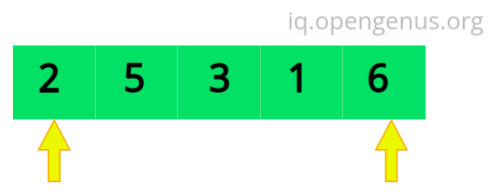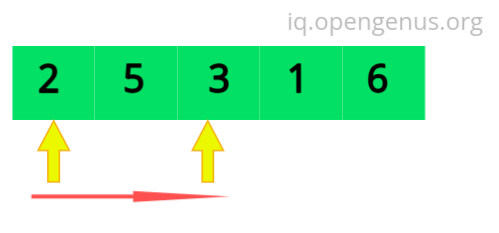×

Search anything:

# Two pointer technique in JavaScript

#### Algorithms JavaScriptGet this book -> Problems on Array: For Interviews and Competitive Programming

Introduction

The two pointer technique is one of the tools to solve competitive programming and to solve technical interviews. We have explained how to use it in JavaScript.

The pattern in two pointer is that by processing two elements over a single loop we can improve the time and space complexity. That is why it is more efficient than the naive approach( Brute force approach ) which processes a single element per loop.

we can apply this approach either on arrays or linked list to find sub elements or a pair which fulfills some condition.

Methods:

1. Two pointers, one starting from the beginning of an array and the other starting from the end until they meet1. One moving at slow pace and the other moving at faster pace.Example Problem 1:
You are given an array people where people[i] is the weight of the ith person, and an infinite number of boats where each boat can carry a maximum weight of limit. Each boat carries at most two people at the same time, provided the sum of the weight of those people is at most limit.

Return the minimum number of boats to carry every given person.

Input: people = [3,2,2,1], limit = 3

we need three boats

• One boat carries The first person which is at index 0 of the array.
• The second one carries only the second person( i.e at index 1) because we our maximum weight limit is three.
• The third one carries the Third person and the 4th person (i.e at index 2 and 3 of the array) because the sum of the two persons weight is equal to the limit.

Algorithm

1. sort the array
2. Initialize two variables(i.e our pointers) called small and big. The first one starts at index 0. The second one starts at the last index of the array.
3. Initialize another variable called boat. which counts the number of boats required to carry the persons.
4. loop trough the array with a condition of small is less than or equal to big.

Explanation

Input: people = [3,2,2,1], limit = 3

1. after the sorting the array becomes [1,2,2,3]
2. [1,2,2,3] -> [1,2,2,3] => boat = 1
3. [1,2,2,3] => boat = 2
4. [1,2,2,3] => boat = 3

Javascript implementation

``````var numRescueBoats = function(people, limit) {
people.sort((a, b) => a - b);
let boat = 0, big = people.length-1;
let small = 0;
while(small<big) {
if(people[small] + people[big] <= limit) {
big--;
small++;
boat++;
}else{
big--;
boat++;
}
}
if(small===big){
boat++;
}
return boat;
};
``````

Time and Space Complexity
The time complexity is O(NlogN+M). The time complexity for sorting the array is O(NlogN) and the time complexity for the two pointer implementation is O(M).
The space complexity is O(1).

Example Problem 2:
Given an integer array nums, move all 0's to the end of it while maintaining the relative order of the non-zero elements.

Note that you must do this in-place without making a copy of the array.

Input: nums = [0,1,0,3,12]

Algorithm

1. declare Two pointers(used as slow runner and fast runner), The slow runner which checks for '0'. The fast runner checks for an element different from '0'.
2. if the fast runner is different from '0', then swap the elements that are at fast runner and at slow runner.

Explanation

Input: nums = [0,1,0,3,12]
The first bold element is at the index of slow runner pointer and the the second bold element is at the fast runner pointer.

1. [0,1,0,3,12] -> [1,0,0,3,12] => shift 0 and 1
2. [1,0,0,3,12] -> [1,0,0,3,12] => the fast runner increment to the next index.
3. [1,0,0,3,12] -> [1,3,0,0,12] => shift 0 and 3
4. [1,3,0,0,12] -> [1,3,12,0,0] => shift 0 and 12

Javascript implementation

``````var moveZeroes = function(nums) {
let p1 = 0;
let p2 = 0;
while(p2<nums.length){
if(nums[p2]!==0){
[nums[p1],nums[p2]] = [nums[p2],nums[p1]];  //swap the two elements
p1++;
}
p2++;
}
};
``````

Time and Space Complexity
We have one loop and no sorting is required the time complexity is O(N).
Space Complexity of O(1).

Example Problem 3:

If there are two middle nodes, return the second middle node.

Algorithm

1. Initialize two pointers. Both of them start from the head of the linked list.
2. The second pointer moves double the speed of the first pointer.
3. When the second pointer reaches the end of the linked list the first pointer reaches the middle of the linked list.
4. return the first pointer.

Explanation

1. [1,2,3,4,5] => The first pointer points at '2' and the second at '4'
2. [1,2,3,4,5].next = null =>the first pointer points at '3' and the second pointer points at next of '5' which is null.
3. return the first pointer, which prints out nodes starting from the middle Node of the linked list.

Javascript implementation

``````    var middleNode = function(head) {
while(p2 !==null && p2.next !== null){
p1 = p1.next;
p2 = p2.next.next;
}
return p1;
};

``````

Time and Space Complexity
The time complexity is linear O(N). The real time performance of the above
implementation is much better than the array.
The space complexity is constant. because the amount of space required is independent of the input parameters

Conclusion
The two pointer method a must have tool to be a good competitive programmer as well as to solve technical interviews. The use of the two pointers is not limited to kind of the above problems. it is also the base for solving sliding window problems. With this article at OpenGenus, you must have the complete idea.#### Abrham Lakew

Electrical and Computer Engineer, Completed Engineer's degree in Electrical and Computer Engineering at Addis Abeba Science and Technology. SDE Intern at OpenGenus.